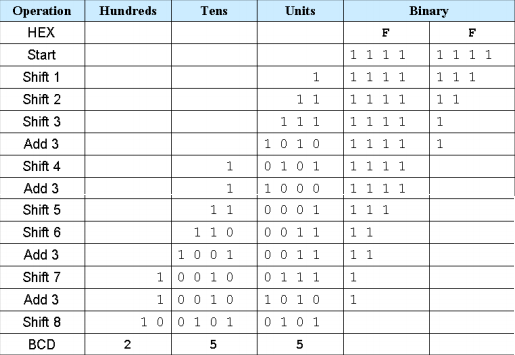<acronym id="xonnx"></acronym>
<td id="xonnx"></td>
1. <pre id="xonnx"></pre>

1. 當前位置：單片機教程網 >> MCU設計實例 >> 瀏覽文章

# verilog實現任意位二進制轉換BCD

作者:kb129   來源:kb129   點擊數:  更新時間：2014年06月08日   【字體：
一直感覺這是個很簡單的問題，直到突然自己連BCD都不太清楚的時候，才發現這沒有看起來那么簡單，這里介紹里任意位二進制轉為BCD的verilog代碼，這個轉換方法也可以用在C上面，此為原創，轉載請注明，謝謝。

基礎知識：

BCD：BCD碼又稱為8421碼，

意義：之所以有時候需要將binary轉換為BCD，一般是用在本科的實驗中，為了將binary顯示在數碼管中，當然還有很多應用，只是目前我還沒有用到。

轉換算法：左移加3法

移位加3法的具體原理，在網上感覺也沒有人能夠說的清楚，以后找到書籍再說吧。下面解釋下左移加三算法。這里以8bit二進制數FF做例子。該算法的操作為上圖。下面對上圖的操作進行詳細的解釋：

注意2：n位的binary就需要進行n次左移

注意4 :  親自推導16位的，和24位的binary轉換，結果正確，所以該算法適用于任意位binary

to  BCD，當然這種論斷沒有足夠的理論依據。

verilog代碼：

說明：對于8bit及以下的binary，可以使用case語句實現移位加三算法。由于這里說明的是任意位的二進制數，轉為BCD，所以我的代碼中設計了一個狀態機，來控制移位，加三和結束操作。由于代碼編寫時間倉促，其中或許有些bug。

//name: 二進制轉BCD
//data: 2014-04-17 at kb129
//info: as 2**8=255  change to BCD then this need 3 times of “8421”
module b_to_bcd(
clk,
rst_n,
binary,
state_en,
BCD
);
parameter   b_length      = 8;
parameter   bcd_len       = 12;
parameter   idle              = 5'b00001;
parameter   shift             = 5'b00010;
parameter   wait_judge   = 5'b00100;
parameter   judge           = 5'b01000;

input       clk;
input       rst_n;
input    [b_length-1:0]  binary;
input      state_en;
output reg [bcd_len-1:0]  BCD;

reg  [b_length-1:0]  reg_binary;
reg  [3:0]    bcd_b, bcd_t, bcd_h;
reg  [3:0]    shift_time;
reg   [5:0]    c_state, n_state;
reg      change_done;
//this is a three section kind of state code style
always@(posedge clk or negedge rst_n)

begin
if(!rst_n)
c_state <= idle;
else
c_state <= n_state;
end
//the second section
always@(posedge clk or negedge rst_n)
begin
if(!rst_n)
c_state <= idle;
else
case(n_state)
idle:begin
if((binary!=0)&&(state_en==1'b1)&&(change_done==0'b0))
n_state <= shift;
else
n_state <= idle;
end
shift: n_state <= wait_judge;
wait_judge: begin
if(change_done==1'b1)
n_state <= idle;
else
n_state <= judge;
end
judge:begin
else
n_state <= shift;
end
n_state <= shift;
end
default: n_state <= idle;
endcase
end
//the third section
always@(posedge clk or negedge rst_n)
begin
if(!rst_n)
begin
shift_time  <= 4'b0;
change_done <= 1'b0;
end
else
case(n_state)
idle:begin
shift_time <= b_length;
reg_binary <= binary;
bcd_h     <= 4'b0;
bcd_t     <= 4'b0;
bcd_b     <= 4'b0;
end
shift:begin
{bcd_h,bcd_t,bcd_b,reg_binary} <= {bcd_h,bcd_t,bcd_b,reg_binary}<<1;
shift_time <= shift_time-1;
if(shift_time==1)     change_done <= 1'b1;
else                       change_done <= 1'b0;
end
wait_judge:begin
if((bcd_h>=4'd5)||(bcd_t>=4'd5)||(bcd_b>=4'd5))
else
if(change_done==1)   BCD <= {bcd_h,bcd_t,bcd_b};
end
if(bcd_h>=4'd5) bcd_h <= bcd_h + 4'b0011; else bcd_h <= bcd_h;
if(bcd_t>=4'd5) bcd_t <= bcd_t + 4'b0011; else bcd_t <= bcd_t;
if(bcd_b>=4'd5) bcd_b <= bcd_b + 4'b0011; else bcd_b <= bcd_b;
end
default: begin
change_done <= 1'b0;
end
endcase
end
endmodule

代碼的仿真：這里對上面的代碼進行了仿真，仿真結果顯示上面代碼可以實現8bitbinary的BCD轉換。

testbench：

module  filter_tb;
reg clk;
reg rst_n;
reg state_en;
reg  [7:0] binary;
wire [11:0] BCD;

initial
begin
clk=0;
rst_n=0;
state_en = 0;
#100   binary = 8'h3f;
#150   rst_n=1;
#200     state_en =1;
end
always  #10  clk=~clk;
b_to_bcd u_b_to_bcd
(
.clk(clk),
.rst_n(rst_n),
.binary(binary),
.state_en(state_en),
.BCD(BCD)
);
endmodule

仿真結果：二進制的3f=十進制的63

關閉窗口

## 相關文章

###### 推薦文章
欧美性色欧美精品视频,99热这里只有精品mp4,日韩高清亚洲日韩精品一区二区,2020国自产拍精品高潮